All-Pole IIR filters

Part 1 of this article first describes generalized formulas for any 2-pole polynomial, no-zero, lowpass or highpass, infinite impulse response (IIR) filter. We then extend the 2-pole filter to a generalization for any even-order all-pole polynomial filter.

Good news for those who want answers without effort: Part 2 of this article shows recipes for constructing some traditional lowpass and highpass IIR filters, as well as tests of these filters.

Part 1. Generalized calculations for any even-pole polynomial lowpass/highpass filter, having no zeros

The filters described in this article are all-pole filters with no zeros. That is, an all-pole filter has a frequency response function that goes infinite (poles) at specific frequencies, but there are no frequencies where the response function is zero. Basically the filter function (also called the transfer function) is a ratio with a constant in the numerator and a polynomial in the denominator. The poles (roots of the polynomial) are located in the negative frequency space, so the actual frequency response (the positive space) is well behaved.

Here we show how to calculate the IIR filter coefficients for the family of filters having two poles (i.e. a second order polynomial in the denominator of the transfer function). Next, we demonstrate how to break down a 4th-order polynomial filter into two different 2-pole stages. This is important if, for example, you want a 4-pole filter of a particular type; for example the properties of a 4-pole Bessel cannot be realized simply by stacking two 2-pole Bessels.

Generalized 2-pole lowpass filter

• First, define the frequency response of the filter as an inverted 2nd-degree polynomial function H(s). This is called the Transfer Function in the s plane, where s is the imaginary axis. The amplitude response versus frequency is a function along the s axis, where s is analogous to the frequency, ranging from −∞ to +∞, with DC at the origin. A generalized 2-pole lowpass transfer function has the form:

H(s) = g ⁄ (s2 + ps + g)

where g is a gain constant causing H(0) = 1, and p is a polynomial coefficient. If you have a coefficient on s2 you can divide the numerator and denominator by that coefficient and absorb it into p and g.

For example, for the specific filters shown in Part 2 below, the transfer functions are:

 Butterworth: H(s) = 1 ⁄ (s2 + √2 s + 1) Critically-damped: H(s) = 1 ⁄ (s2 + 2s + 1) Bessel: H(s) = 3 ⁄ (s2 + 3s + 3)

If you only know the complex roots (α ± ) of your 2nd-degree polynomial in the denominator (which usually means the constants g and p aren't nice clean integers), then use

p = −2α
g = α2 + β2

• Next, we calculate the cutoff frequency correction factor c. To do this, we substitute for s in H(s), where j=√−1. Then, we multiply this by its complex conjugate (i.e. substituting −j for j) to get the squared amplitude response:

|H(ω)|2   =   H() H(−)   =   g2 ⁄ (ω4 − (2gp2)ω2 + g2)

Cascading the filters n times is equivalent to raising |H(ω)|2 to the n-th power. Because we want the 3 dB cutoff frequency (where the squared amplitude is 0.5) to be the same for any number of cascades, simply set |H(ω)|2n = ½ and solve for ω. Because |H(ω)|2 is a quadratic function of ω2, we use the quadratic formula to solve for ω2. The correction factor c is simply the inverse of the square root of the result. In other words:

c2   =   1 ⁄ ω2   =   2 ⁄ { 2gp2 + [ (2gp2)2 − 4g2(1 − 21⁄n) ]½ }

Take the square root of the above to get the correction factor c, which we apply to the cutoff frequency f0 in the next step, to preserve the 3 dB cutoff position for any number of n filter passes.

• Because this is an analog filter, we need to make another adjustment to the cutoff frequency, to "warp" it from the analog world to the digital world. For the rest of the calculations, we will use the adjusted digital cutoff frequency ω0:

ω0 = tan(π c f0fs)

where f0 is the analog cutoff frequency and fs is the sampling frequency.

• Now we need the coefficients of the filter. To do this, we perform a bilinear transformation from the s domain to the z domain. This isn't as hard as it sounds; it's just algebraic manipulation. Basically it involves substituting s = (z−1) ⁄ [ω0(z+1)] in H(s), collecting the powers of 1 ⁄ z together in the numerator and denominator, normalizing things so that the coefficient for z0 in the denominator is 1, and reading off the coefficients. The ai coefficients (which are multiplied by the most recent xi inputs) will be in the numerator and the bi coefficients (which are multiplied by the most recent yi outputs) will be their negative in the denominator. After simplifying, the coefficients are:

a0 = g ω02 ⁄ (1 + p ω0 + g ω02)
a1 = 2a0
a2 = a0
b1 = 2a0 (1 ⁄ (02) − 1)
b2 = 1 − (a0 + a1 + a2 + b1)

• Finally, calculate the filter:

yk = a0 xk + a1 xk−1 + a2 xk−2 + b1 yk−1 + b2 yk−2

Generalized 2-pole highpass filter

To get a highpass filter, use ω0 = 1 ⁄ tan[πf0 ⁄ (cfs)] as the adjusted digital cutoff frequency (that is, invert c before applying to f0 and invert the tangent to get ω0), and calculate the lowpass coefficients. Then, negate the coefficients a1 and b1 — but be sure you calculate b2 before negating those coefficients! Applying these coefficients in the final filter formula above, will result in a highpass filter with a 3 dB cutoff at f0.

Generalized 4-pole filter

A 4th-order polynomial filter has a transfer function of the form:

H(s) = g ⁄ (s4 + ps3 + qs2 + rs + g)

Although we can perform the same bilinear transformation here as with the 2nd-order filter, and obtain a 1-pass 4-th order filter, we'll run into trouble if we do this. We'd find that the filter coefficients may have magnitudes different by several orders (say, one coefficient might be 0.013 and another might be 128.2), which will result in instability due to the finite precision of the computer. Using double precision helps, but the real solution is to decompose the 4th-order filter into a cascade of two 2nd-order filters.

Usually, a 4th-order polynomial cannot be factored readily into two clean 2nd-order polynomials. However, we know that the polynomal can be rewritten in terms of its roots; i.e. the four values s = {R1, R2, R3, R4} that cause the polynomial to equal zero. Therefore the transfer function becomes:

H(s) = g ⁄ [ (sR1) (sR2) (sR3) (sR4) ]

...and we know that g is the product of all the roots; i.e. g = R1R2 R3R4.

We can calculate the complex roots of a 4th-order polynomial using a number of online tools. For example, SymbMath by Dr. Weiguang Huang is a free web-based math solver, implemented as a Java applet, that will solve for the roots of a 4th-order polynomial (in the Run menu, select "Solve Polynomial").

Let's use the 4-pole Bessel transfer function as an example:

n pole Bessel:   H(s) = g ⁄ (p0 + p1s + p2s2 + ... + pnsn),   pi = (2ni)! ⁄ [ 2ni i! (ni)! ],   g = p0
4 pole Bessel:   H(s) = 105 ⁄ (s4 + 10s3 + 45s2 + 105s + 105)

SymbMath shows that the four roots of the denominator are:

R1, R2  =  α1 ± 1  =  −2.1037893971796278 ± 2.657418041856753j
R3, R4  =  α2 ± 2  =  −2.8962106028203722 ± 0.8672341289345028j

Setting g1 = R1R2 = α12 + β12 and g2 = R3R4 = α22 + β22 and combining the corresponding root pairs in the denominator of H(s), we get a product of two 2nd-order transfer functions:

H(s)   =   g1 ⁄ (s2 − 2α1s + g1)   ×   g2 ⁄ (s2 − 2α2s + g2)

We set p = −2α and solve for the coefficients of each 2-pole filter stage separately, as described in the previous section.

Our remaining problem involves the frequency correction factor c, which must be the same for each stage. This must be calculated, as with the 2-pole filter, by solving for ω in H()H(−jω) = ½. This is a quartic function of ω2, and unlike quadratics, quartics are non-trivial to solve. SymbMath can solve them. For a 4-pole Bessel lowpass filter, the correction factor c = 1 ⁄ ω = 1 ⁄ 2.1139176749042.

After all this, the 4-pole filter is then realized by feeding the input data into the first stage, and feeding that output into the second stage.

Note that the two 2nd-order filter stages making the whole 4th-order filter are different. That's why cascading two identical 2-pole filters won't result in a 4-pole filter of the same type. That is, if you want a 4th-order Bessel, you can't start out by cascading two 2nd-order Bessels; you must instead decompose the 4th-order Bessel into two different 2nd-order filters (which aren't themselves Bessels).

Any even-order filter can be decomposed into a cascade of 2-pole filters in the same manner described above, although for higher-order filters you will likely have to resort to numerical approximation techniques to solve for the polynomial coefficients and for the frequency correction factor.

Part 2. Recipes for 2-Pole IIR Filters

If you print this, you may want to do so in landscape mode so that the width of the table fits on the page.

You can also download an Excel spreadsheet (74 kilobytes) that demonstrates the generalized 2-pole polynomial filter. You can play with the g and p polynomial coefficients to see how the filter reacts to an input signal consisting of a square wave impulse followed by Gaussian noise.

Calculation steps:

 Definitions n = number of filter passes L0 = 3 dB cutoff wavelength f0 = 1 ⁄ L0 = 3 dB cutoff frequency fs = sample frequency xk = input value at index k yk = filter output value at index k Filter type Low Pass High Pass Butterworth Critically Damped Bessel Butterworth Critically Damped Bessel 3 dB cutoffcorrection c = (21⁄n − 1)−¼ c = (21/(2n) − 1)−½ c = [(21⁄n − ¾)½ − ½]−½⁄ √3 c = (21⁄n − 1)¼ c = (21/(2n) − 1)½ c =√3 [(21⁄n − ¾)½ − ½]½ polynomialcoefficients g = 1 p = √2 g = 1 p = 2 g = 3 p = 3 g = 1 p = √2 g = 1 p = 2 g = 3 p = 3 correctedcutoff frequency f* = c f0 ⁄ fs f* = ½ − c f0 ⁄ fs ensure fs is OK forstability constraint 0 < f* < 1⁄8 (unstable when f* ≥ 1⁄4) 3⁄8 < f* < 1⁄2 (unstable when f* ≤ 1⁄4) warp cutofffreq from analogto digital domain ω0 = tan(π f*) filtercoefficientcalculations K1 = p ω0 K2 = g ω02 A0 = K2 ⁄ (1 + K1 + K2) A1 = 2A0 A2 = A0 B1 = 2A0 (1 ⁄ K2 − 1) B2 = 1 − (A0 + A1 + A2 + B1) filtercoefficients a0 = A0 a1 = A1 a2 = A2 b1 = B1 b2 = B2 a0 = A0 a1 = −A1 a2 = A2 b1 = −B1 b2 = B2 filter (1 pass) yk = a0 xk + a1 xk−1 + a2 xk−2 + b1 yk−1 + b2 yk−2

Notes on the 3 filters

• As shown in the table above, a highpass filter is constructed from a lowpass filter by inverting c and ω0, and negating the lowpass coefficients a1 and b1. Note that the identity 1 ⁄ tan(πx) = tan[π(½−x)] is used above to accomplish the inversion of ω0 for the high-pass filter.

• The Bessel has the most linear phase response, with a stopband frequency response midway between the Butterworth and critically-damped filters. See the figure below.

• Cascading n 2-pole Butterworth filters will not yield a 2n-pole Butterworth filter. The same is true for Bessel. Cascading two Butterworths, for example, results in what is known as a "Linkwitz-Riley" filter.

• On the other hand, cascading critically-damped filters will result in another critically-damped filter.

• You can cancel the overshoot of a Butterworth with the lag of the critically-damped filter to obtain another critically-damped filter that has the positive features of both. For example, a Butterworth of cutoff frequency 2f0 cascaded with a two critically-damped filters of cutoff f0 will result in a critically-damped filter that has less lag than the original critically-damped filter cascaded 3 times, yet greater stopband attenuation (steeper falloff). Similarly, an arithmetic average of a 1-pass 2f0 Butterworth and a 2-pass critical filter of cutoff f0, will result in a critically-damped filter having a frequency response similar to the 1-pass Butterworth (i.e. better than a 1-pass critical), and with a lag in between the Butterworth and the 2-pass critical.

Tests

Frequency Response

The following frequency response curves show the performance of each filter above, for different combinations of cutoff frequency and number of filters cascaded together (passes). These plots also demonstrate that the cutoff frequency correction c above does indeed cause the 3 dB cutoff frequency to occur in the same place for each filter, regardless of the number of passes.

The frequency responses shown resulted from using a chirp as the input signal (i.e., a sinewave that increases in frequency over time). The chirp contained 32,000 data points and covered over 5 octaves, with the wavelength 1 ⁄ f decreasing linearly to allow each frequency to have nearly the same number of cycles approximating that frequency. The plotted curves are maximum squared-amplitude output levels, updated each cycle. The curves look noisy at the short wavelengths (high frequencies) due to the chirp wavelength nearing the Nyquist limit of 2, resulting in inconsistent wave amplitudes in each cycle. The sampling frequency fs = 1 in all cases.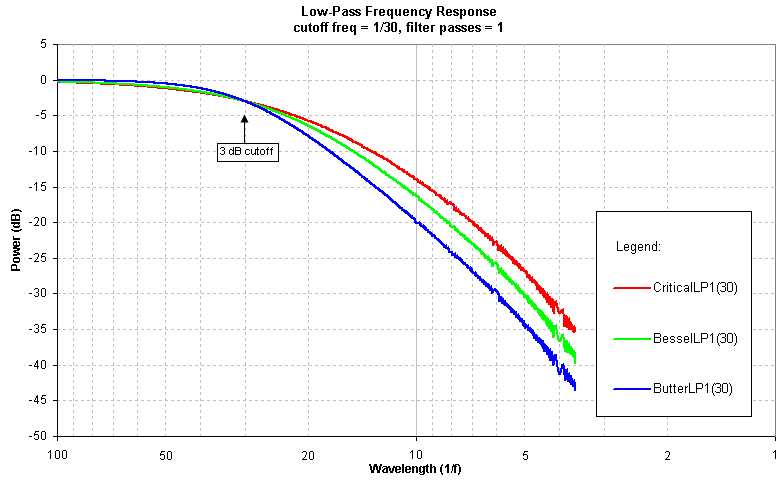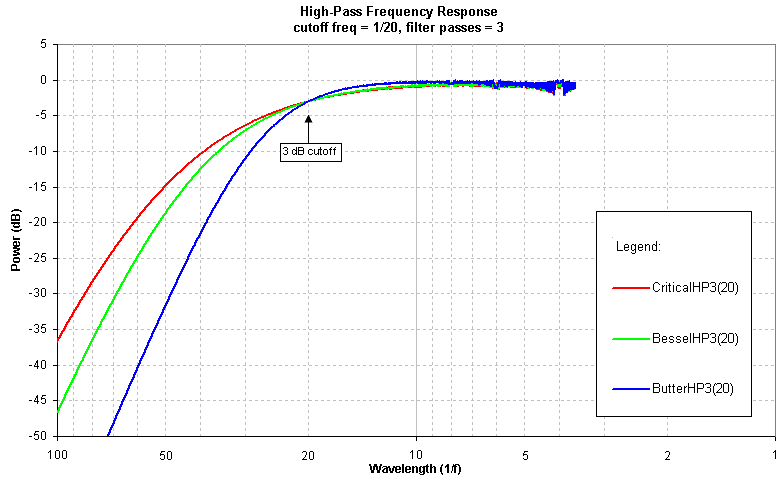Temporal Response to a Step Function

The following time-domain responses to a step function show the performance of each filter, for the same cutoff frequency (1/80 in this case), plotted on the same time scale, for different number of passes. We make the following observations:

• All high-pass filters, even when critically damped, will oscillate. For a step response one can generally expect a critically-damped filter to exhibit one less zero-crossing than the number of poles in the filter (and the more one cascades filters, the more poles one has). Underdamped filters like the Butterworth will oscillate more.

• The critically-damped filter has the fastest rise time, yet the Bessel appears to converge faster.

• The Bessel actually has a microscopic amount of overshoot, so that it appears to settle faster than the critically-damped filter, however the amplitude of the Bessel's oscillations in the highpass case are higher than the critically-damped filter.

• Although the Butterworth filter has the flattest passband response, sharpest rolloff, and steepest falloff of all the filters shown, in the time domain it suffers from overshoot. The lowpass Butterworth also exhibits the most lag.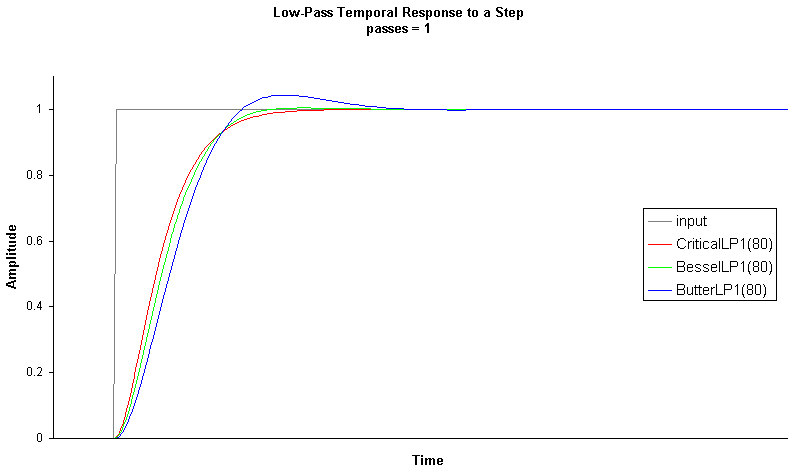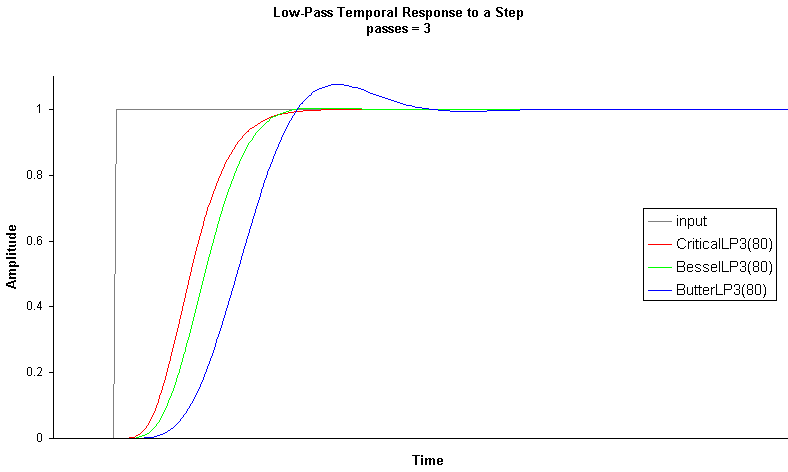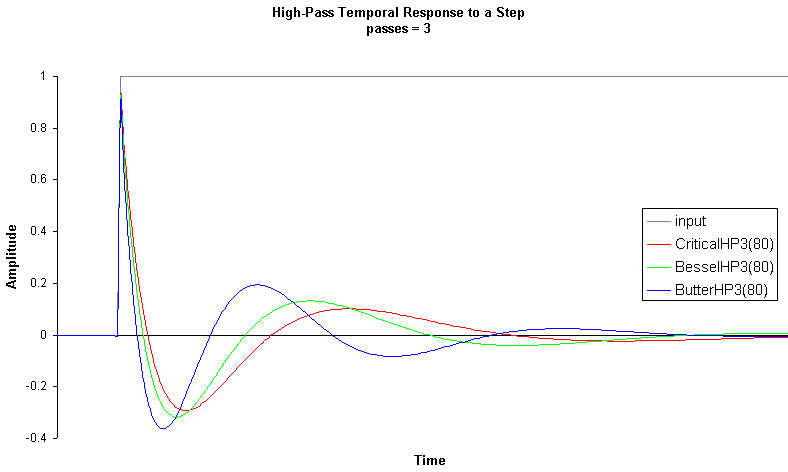References:

Young-Hoo Kwon, Butterworth Digital Filters

D. Gordon E. Robertson and James J. Dowling, "Design and responses of Butterworth and critically damped digital filters," Journal of Electromyography and Kinesiology 13 (2003) pp 569-573.

S.D. Murphy and D.G.E. Robertson, "Construction of a High-pass Digital Filter," presentation to the North American Conference on Biomechanics II (CSB VII), Chicago, USA, 1992.

Peter Nachtwey, Bessel High Pass

R.W. Erickson, Filter Circuits, 10 March 1999.

Usenet thread in comp.dsp, "Wanted: critically-damped high-pass IIR filter."